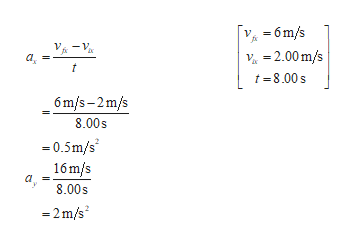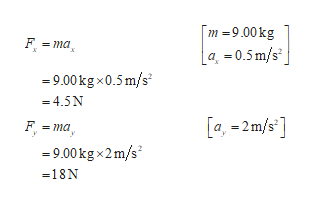A toy rocket engine is securely fastened to a large puck that can glide with negligible friction over a horizontal surface, taken as the xy plane. The 9.00-kg puck has a velocity of 2.00 î m/s at one instant. Eight seconds later, its velocity is (6 î + 16 ĵ) m/s.a) Assuming the rocket engine exerts a constant horizontal force, find the components of the force.b) Find its magnitude.

Question

A toy rocket engine is securely fastened to a large puck that can glide with negligible friction over a horizontal surface, taken as the xy plane. The 9.00-kg puck has a velocity of 2.00 î m/s at one instant. Eight seconds later, its velocity is (6 î + 16 ĵ) m/s.

a) Assuming the rocket engine exerts a constant horizontal force, find the components of the force.

b) Find its magnitude.

Step 1

The initial velocity of the puck is 2.00im/s, and the final velocity is (6i+16j)m/s.

(a)Write the expression for the components of velocity.help_outlineImage Transcriptionclose-6m/s -1 -2.00m/s a t t-8.00s 6m/s-2m/s 8.00s 0.5m/s 16 m/s a 8.00s -2m/s fullscreen
Step 2

Write the expression for the horizontal component, and vertical component of velocity.help_outlineImage Transcriptionclosem -9.00kg F ma a =0.5m/s 9.00kg x0.5 m/s =4.5N [a,-2 m/s] F ma 9.00kgx2m/s -18N fullscreen
Step 3

Hence the expression for fo...

Want to see the full answer?

See Solution

Want to see this answer and more?

Our solutions are written by experts, many with advanced degrees, and available 24/7

See Solution
Tagged in

Physics